# 14. Networking and communications¶

This week was Networking and communications and these were the assignments

## Assignments¶

Individual assignment:

• Design, build, and connect wired or wireless node(s) with network or bus addresses.

Group assignment:

• Send a message between two projects.

## Research¶

This week Everything I did was pretty straight forward, I redrew Neils’ board of Asynchronous serial bus and downloaded the C file and makefile and programmed the board.

## Board Design¶

As mentioned earlier I redrew the boards, there are two board designs one board has FTDI which is called a Bridge and other board has no FTDI which is called Node.

Bridge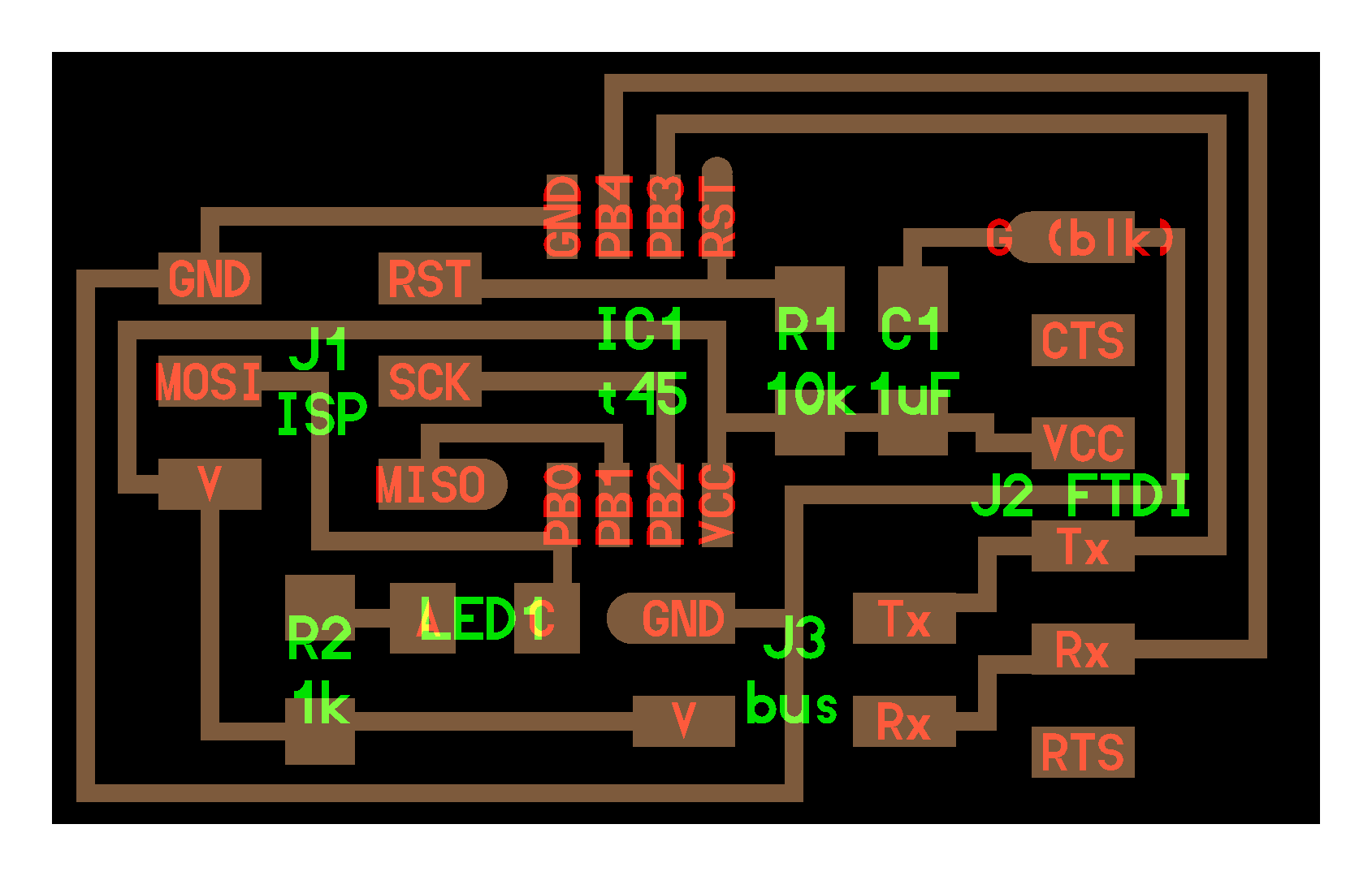Node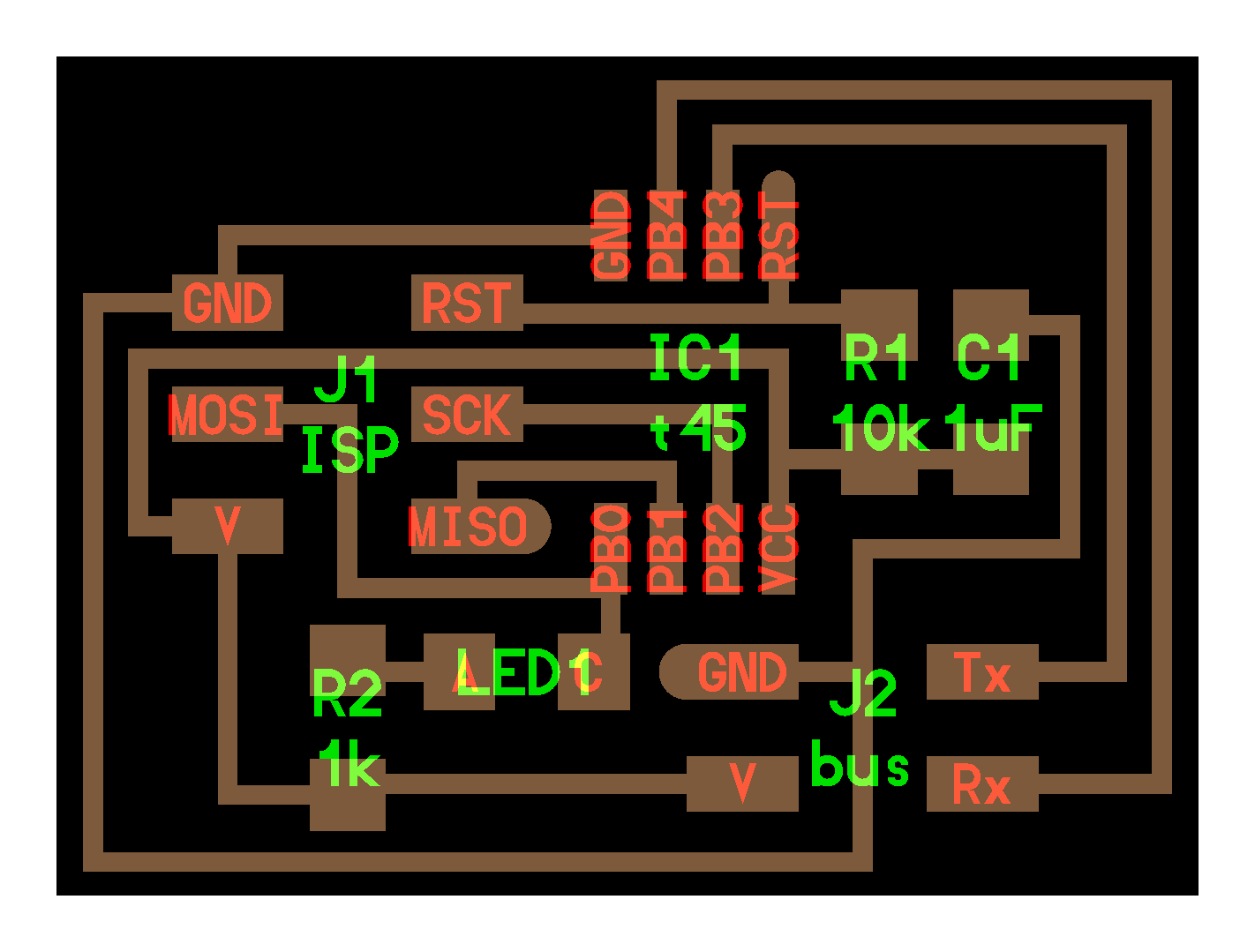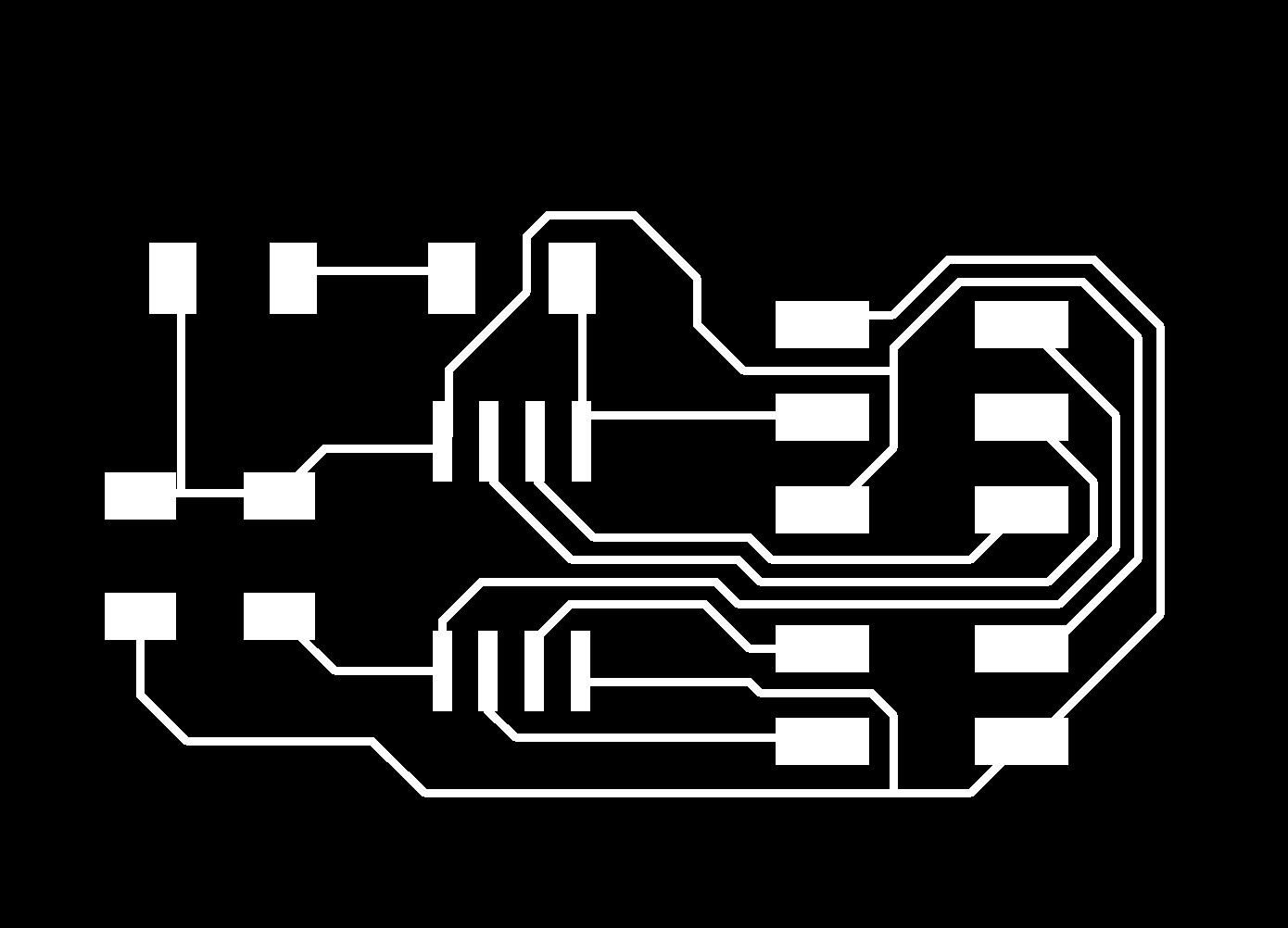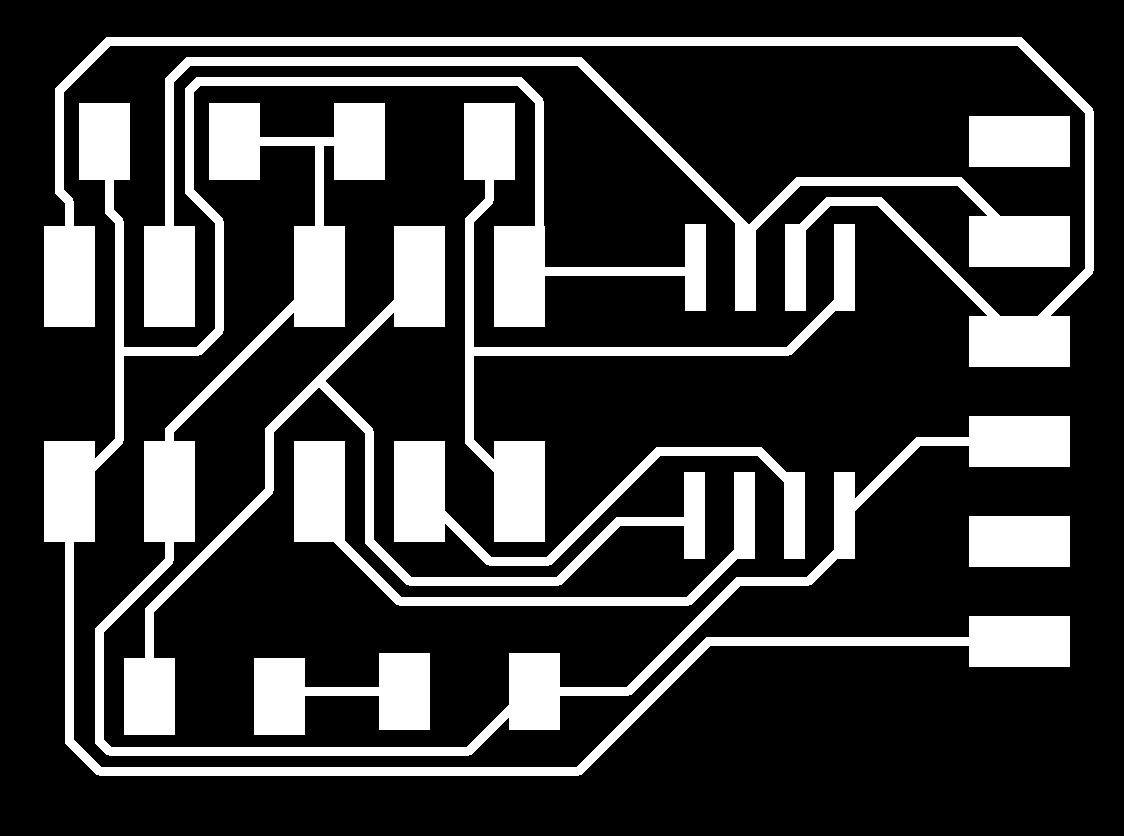Finished Boards: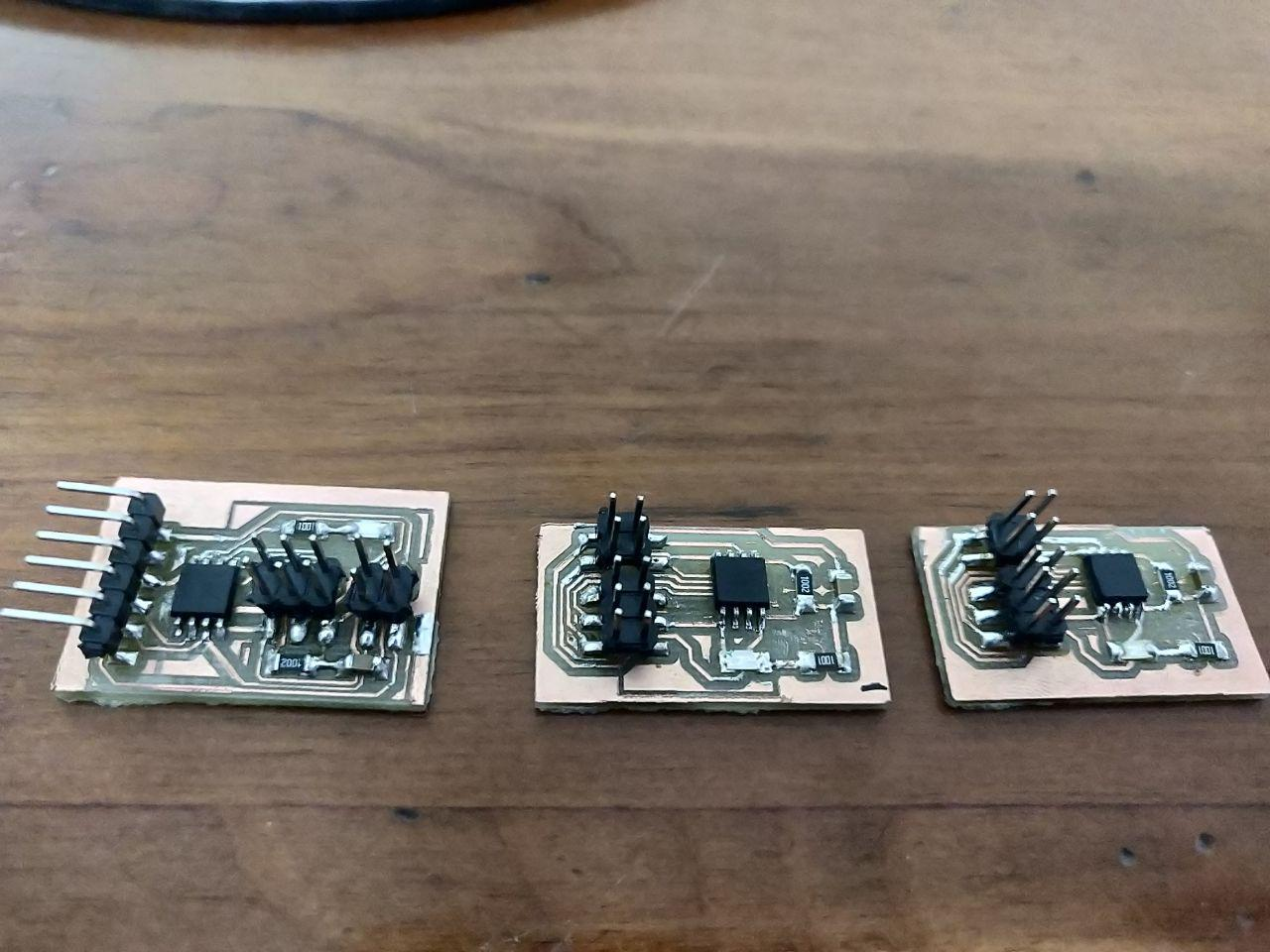## Programming¶

For the two boards, we have to Program them individually with minor tweaks in the code(C file) and when I say minor only one Line has to be changed. In the line `#define node_id '1'` we have to change the number from ‘1’ to ‘0’ while we are programming the Bridge board, likewise the first node board will be numbered ‘1’ and will increase numerically.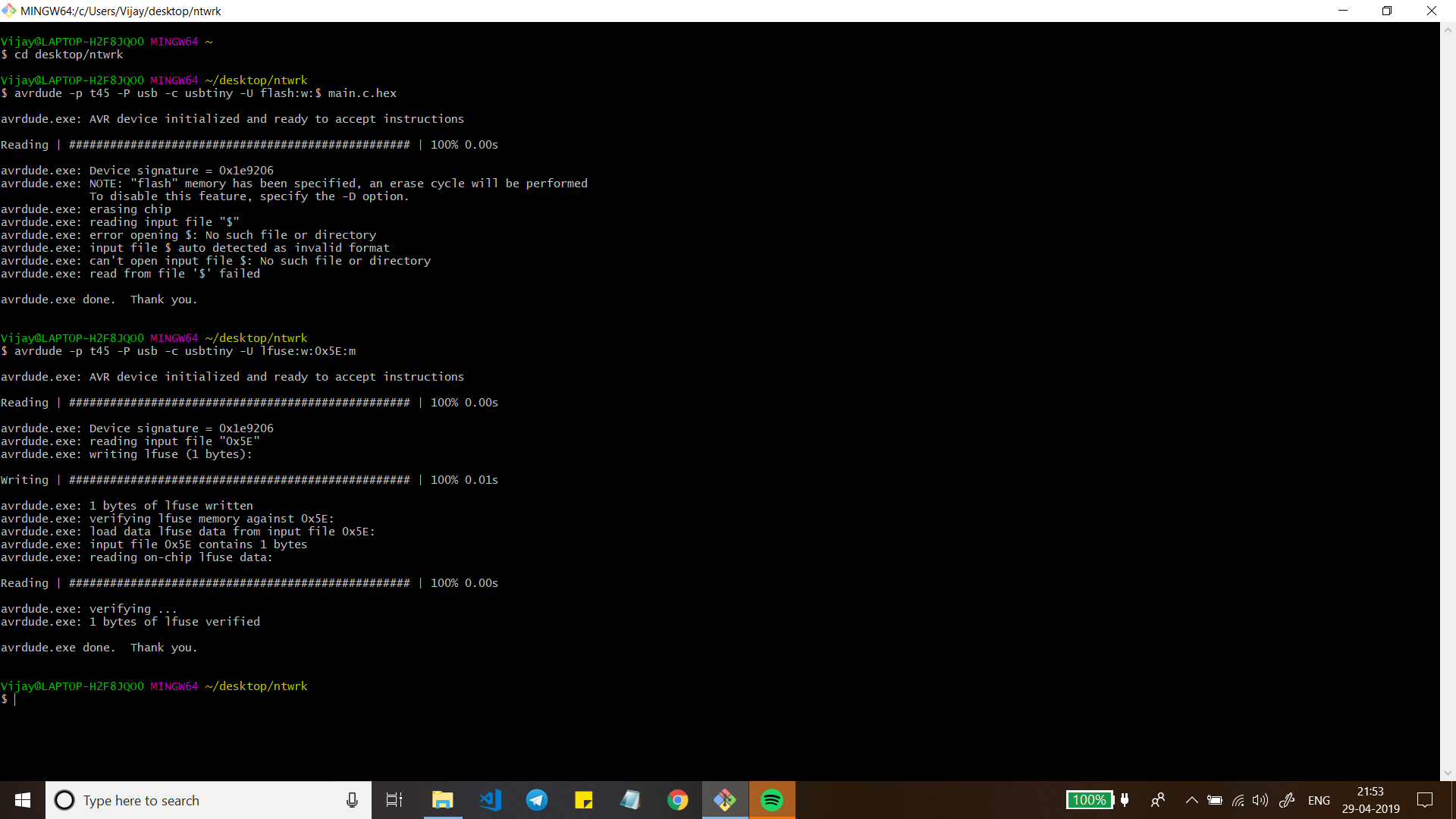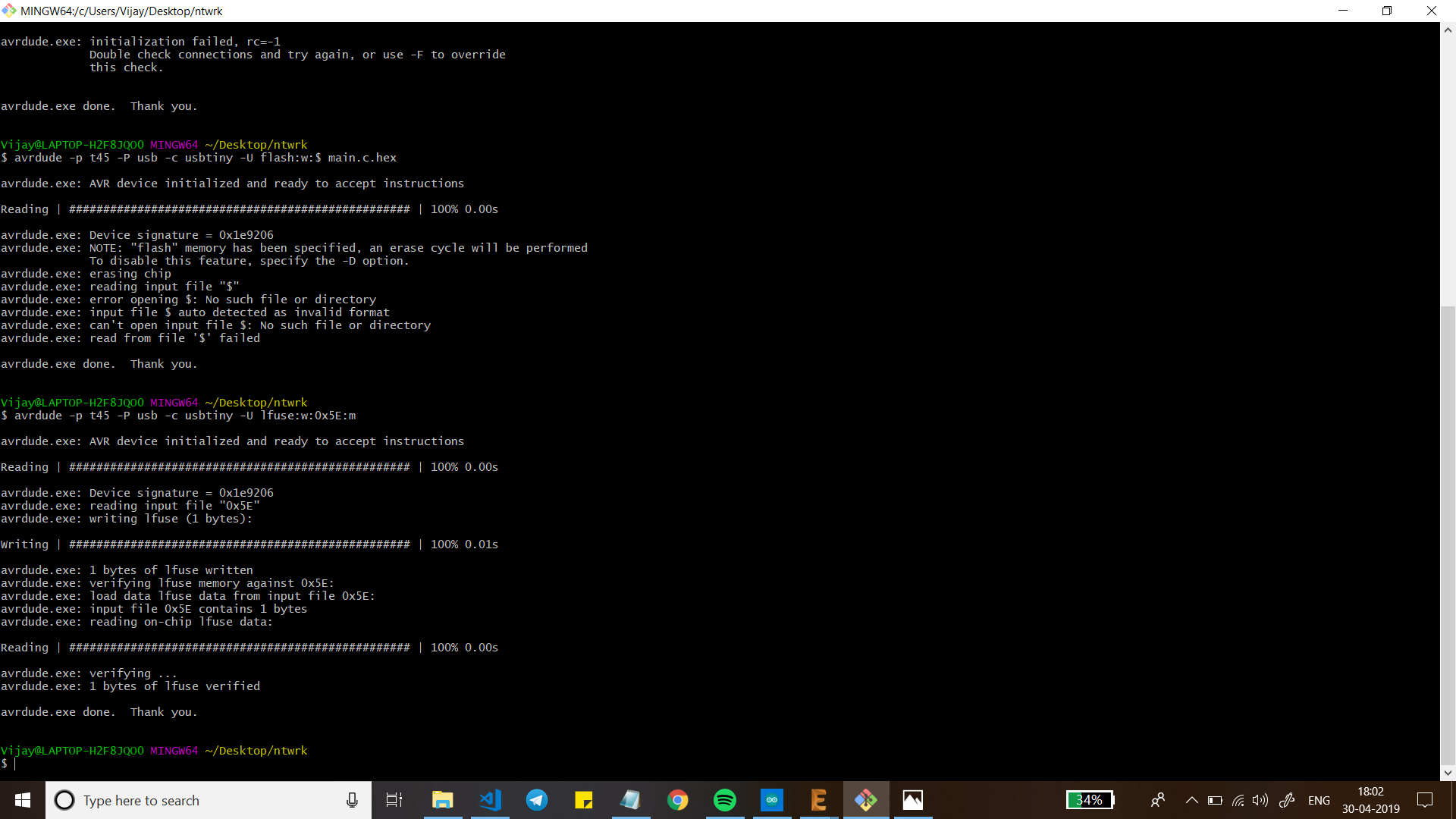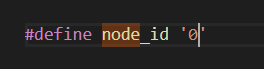### Code¶

C source file:

```//
//
// hello.bus.45.c
//
// 9600 baud serial bus hello-world
//
// Neil Gershenfeld
// 11/24/10
//
// (c) Massachusetts Institute of Technology 2010
// Permission granted for experimental and personal use;
// license for commercial sale available from MIT.
//

#include <avr/io.h>
#include <util/delay.h>
#include <avr/pgmspace.h>
#include <string.h>

#define output(directions,pin) (directions |= pin) // set port direction for output
#define input(directions,pin) (directions &= (~pin)) // set port direction for input
#define set(port,pin) (port |= pin) // set port pin
#define clear(port,pin) (port &= (~pin)) // clear port pin
#define pin_test(pins,pin) (pins & pin) // test for port pin
#define bit_test(byte,bit) (byte & (1 << bit)) // test for bit set
#define bit_delay_time 100 // bit delay for 9600 with overhead
#define bit_delay() _delay_us(bit_delay_time) // RS232 bit delay
#define half_bit_delay() _delay_us(bit_delay_time/2) // RS232 half bit delay
#define led_delay() _delay_ms(100) // LED flash delay

#define led_port PORTB
#define led_direction DDRB
#define led_pin (1 << PB0)

#define serial_port PORTB
#define serial_direction DDRB
#define serial_pins PINB
#define serial_pin_in (1 << PB3)
#define serial_pin_out (1 << PB4)

#define node_id '1'

void get_char(volatile unsigned char *pins, unsigned char pin, char *rxbyte) {
//
// read character into rxbyte on pins pin
//    assumes line driver (inverts bits)
//
*rxbyte = 0;
while (pin_test(*pins,pin))
//
// wait for start bit
//
;
//
// delay to middle of first data bit
//
half_bit_delay();
bit_delay();
//
// unrolled loop to read data bits
//
if pin_test(*pins,pin)
*rxbyte |= (1 << 0);
else
*rxbyte |= (0 << 0);
bit_delay();
if pin_test(*pins,pin)
*rxbyte |= (1 << 1);
else
*rxbyte |= (0 << 1);
bit_delay();
if pin_test(*pins,pin)
*rxbyte |= (1 << 2);
else
*rxbyte |= (0 << 2);
bit_delay();
if pin_test(*pins,pin)
*rxbyte |= (1 << 3);
else
*rxbyte |= (0 << 3);
bit_delay();
if pin_test(*pins,pin)
*rxbyte |= (1 << 4);
else
*rxbyte |= (0 << 4);
bit_delay();
if pin_test(*pins,pin)
*rxbyte |= (1 << 5);
else
*rxbyte |= (0 << 5);
bit_delay();
if pin_test(*pins,pin)
*rxbyte |= (1 << 6);
else
*rxbyte |= (0 << 6);
bit_delay();
if pin_test(*pins,pin)
*rxbyte |= (1 << 7);
else
*rxbyte |= (0 << 7);
//
// wait for stop bit
//
bit_delay();
half_bit_delay();
}

void put_char(volatile unsigned char *port, unsigned char pin, char txchar) {
//
// send character in txchar on port pin
//    assumes line driver (inverts bits)
//
// start bit
//
clear(*port,pin);
bit_delay();
//
// unrolled loop to write data bits
//
if bit_test(txchar,0)
set(*port,pin);
else
clear(*port,pin);
bit_delay();
if bit_test(txchar,1)
set(*port,pin);
else
clear(*port,pin);
bit_delay();
if bit_test(txchar,2)
set(*port,pin);
else
clear(*port,pin);
bit_delay();
if bit_test(txchar,3)
set(*port,pin);
else
clear(*port,pin);
bit_delay();
if bit_test(txchar,4)
set(*port,pin);
else
clear(*port,pin);
bit_delay();
if bit_test(txchar,5)
set(*port,pin);
else
clear(*port,pin);
bit_delay();
if bit_test(txchar,6)
set(*port,pin);
else
clear(*port,pin);
bit_delay();
if bit_test(txchar,7)
set(*port,pin);
else
clear(*port,pin);
bit_delay();
//
// stop bit
//
set(*port,pin);
bit_delay();
//
// char delay
//
bit_delay();
}

void put_string(volatile unsigned char *port, unsigned char pin, PGM_P str) {
//
// send character in txchar on port pin
//    assumes line driver (inverts bits)
//
static char chr;
static int index;
index = 0;
do {
put_char(&serial_port, serial_pin_out, chr);
++index;
} while (chr != 0);
}

void flash() {
//
// LED flash delay
//
clear(led_port, led_pin);
led_delay();
set(led_port, led_pin);
}

int main(void) {
//
// main
//
static char chr;
//
// set clock divider to /1
//
CLKPR = (1 << CLKPCE);
CLKPR = (0 << CLKPS3) | (0 << CLKPS2) | (0 << CLKPS1) | (0 << CLKPS0);
//
// initialize output pins
//
set(serial_port, serial_pin_out);
input(serial_direction, serial_pin_out);
set(led_port, led_pin);
output(led_direction, led_pin);
//
// main loop
//
while (1) {
get_char(&serial_pins, serial_pin_in, &chr);
flash();
if (chr == node_id) {
output(serial_direction, serial_pin_out);
static const char message[] PROGMEM = "node ";
put_string(&serial_port, serial_pin_out, (PGM_P) message);
put_char(&serial_port, serial_pin_out, chr);
put_char(&serial_port, serial_pin_out, 10); // new line
led_delay();
flash();
input(serial_direction, serial_pin_out);
}
}
}
```

Makefile:

```PROJECT=main
SOURCES=\$ main.c
MMCU=attiny45
F_CPU = 20000000

CFLAGS=-mmcu=\$(MMCU) -Wall -Os -DF_CPU=\$(F_CPU)

\$ main.hex: \$ main.out
avr-objcopy -O ihex \$ main.out \$ main.c.hex;\
avr-size --mcu=\$(MMCU) --format=avr \$ main.out
\$ main.out: \$(SOURCES)
avr-gcc \$(CFLAGS) -I./ -o \$ main.out \$(SOURCES)
program-bsd: \$ main.hex
avrdude -p t45 -c bsd -U flash:w:\$ main.c.hex

program-dasa: \$ main.hex
avrdude -p t45 -P /dev/ttyUSB0 -c dasa -U flash:w:\$ main.c.hex

program-avrisp2: \$ main.hex
avrdude -p t45 -P usb -c avrisp2 -U flash:w:\$ main.c.hex

program-avrisp2-fuses: \$ main.hex
avrdude -p t45 -P usb -c avrisp2 -U lfuse:w:0x5E:m

program-usbtiny: \$ main.hex
avrdude -p t45 -P usb -c usbtiny -U flash:w:\$ main.c.hex

program-usbtiny-fuses: \$ main.hex
avrdude -p t45 -P usb -c usbtiny -U lfuse:w:0x5E:m

program-dragon: \$ main.hex
avrdude -p t45 -P usb -c dragon_isp -U flash:w:\$ main.c.hex

program-ice: \$ main.hex
avrdude -p t45 -P usb -c atmelice_isp -U flash:w:\$ main.c.hex
```

## Execution¶

Now the board will be connected in series and we then open Arduino IDE and run the serial monitor. In the serial monitor When we enter the Board ID initially the LEDs in all the boards will light up but the board whose ID we entered will blink again after a slight delay.

## Problems¶

It would be a lie if I said there were no problems that I faced this week so here is a list 1. Board milling. 2. Initializing Failed rc=-1. 3. MakeFile.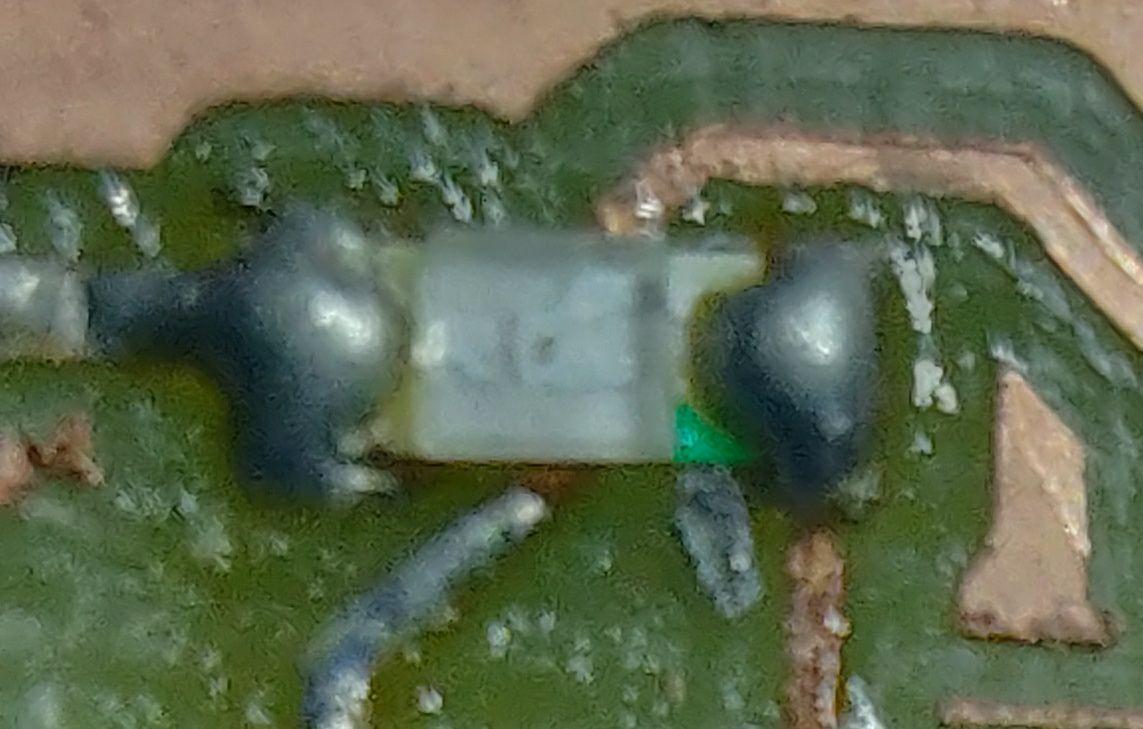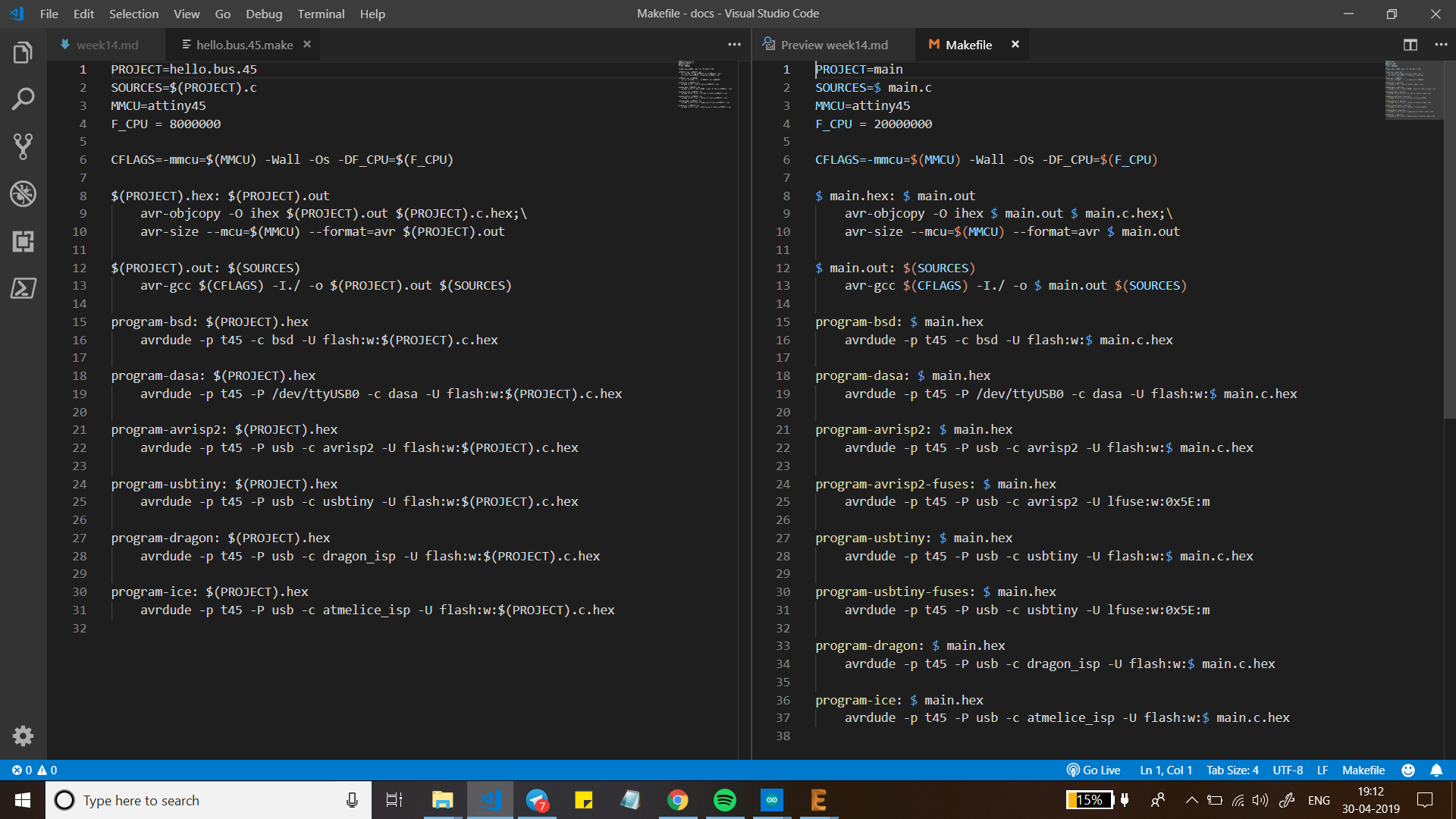## Solutions¶

1. For the first problem I have to confess there were many issues within it like DRC, Thin traces, Shorts and leftover copper layer post milling board(which was the reason for the second problem) I took the help of my instructor and cleaned the boards.
2. For this timeless classic which can take hours together to resolve, I took my instructors help to resolve it luckily this time it took less than the usual amount of time we solved it by resoldering a few components and removing some shorts that were made during stuffing the board(My Bad).
3. This was Interesting as this was a first when I downloaded the MakeFile I couldn’t notice anything wrong with the code but the key giveaway for this problem was I observed that When I opened the file in Visual studio Code the whole code had while color text which meant something was off so When I asked my Instructor what to do he suggested me to take different makefile for the ATTiny45 and run the makefile. The problem was resolved.

## Videos¶

### Coming up…¶

We will be using bluetooth module HC005